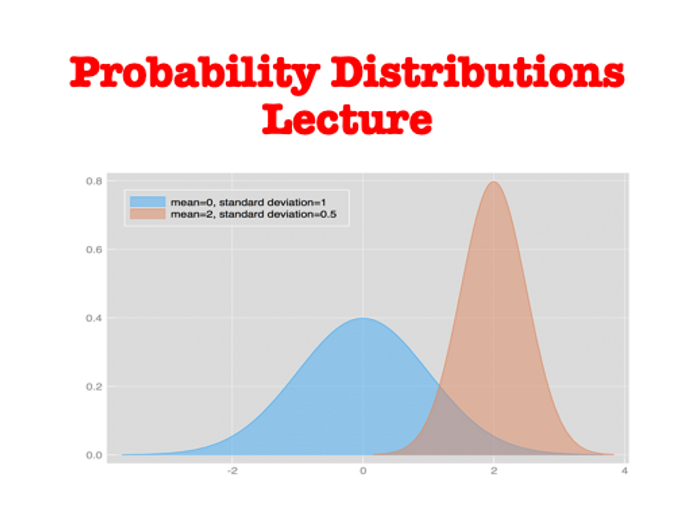Probability Distributions Lecture (Elementary Statistics Module)

This is a lecture which is covered during Elementary Statistics Module with students who just started to learn Statistics.
The main objective of this chapter is to cover Probability Distribution, Binominal Probability Distribution as well as Poisson Probability distribution. More details of the lecture agenda could be found bellow.

Agenda of Introduction to Statistics Lecture is as following:

1. Probability Distribution
Define random variable and probability distribution.
Determine when a potential probability distribution actually satisfies the necessary requirements.
Given a probability distribution, compute the mean and standard deviation, then use those results to determine whether results are significantly low or significantly high.

2. Binominal Probability Distributions
Describe a binominal probability distribution and find probability values for a binominal distribution.
Compute the mean and standard deviation for a binominal distribution, then use those results to determine whether results are significantly low or significantly high.

3. Poisson Probability Distributions
Describe a Poisson probability distribution and find probability values for a Poisson distribution.

By purchasing this teaching resources, you will get:
Probability Distributions Lecture Power Point Presentation
File with the activities for all parts of the lecture agenda. Those activities might be used within seminar or lecture on Probability Distributions.
Test bank to Probability Distributions with all answers to them in order to assess your students. (Overall there are 3 Variants of tests with different questions and all answers to them)

Please Write comments as well as subscribe to our store!

\$9.12
Save for later

Info

Created: Sep 18, 2019

Whole lesson

zip, 2 MB

Probability-Distributions-Lecture

Report a problem

Tes Paid Licence

How can I re-use this?